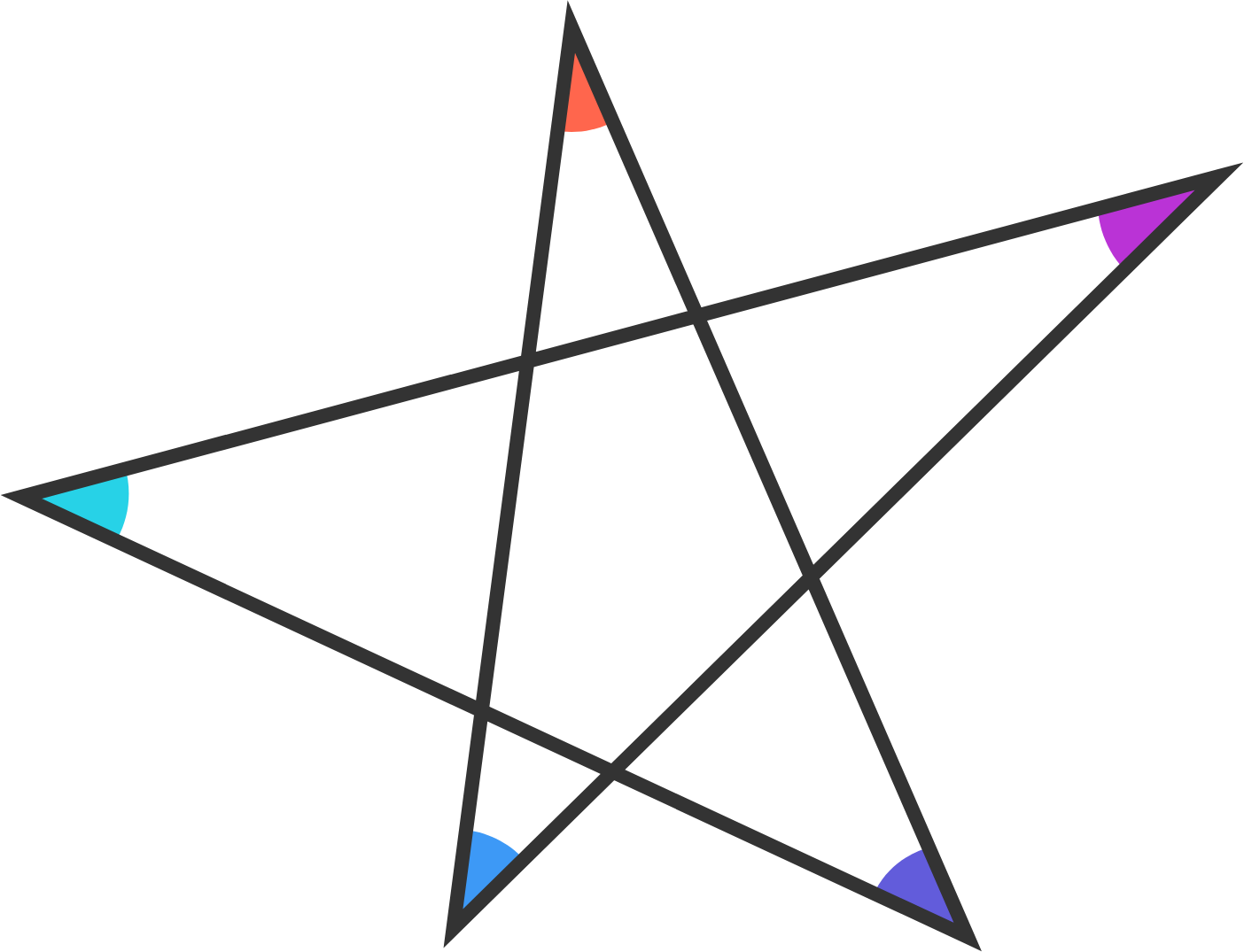# Problems of the Week

Contribute a problem

# 2017-07-03 Basic

If $m$ is odd and $n$ is even, which of the following is definitely an even number?

A. $\ m^2 + n$
B. $\ m^2 + mn$
C. $\ m^2+n^2$
D. $\ m+ n^2$
E. $\ mn + n^2$

Find $n$: $1!\times2!\times3!\times\cdots\times n! = 125,411,328, {\color{#3D99F6}000}.$


Notation: $!$ is the factorial notation. For example, $8! = 1\times2\times3\times\cdots\times8$.

What is the sum of the measures of the colored angles?A magnifying glass is a convex lens. It makes a nearby object look bigger by converging light rays.

Now suppose you look at the same object with the magnifying glass while underwater, keeping the distance between the object and the lens fixed. How would it appear?

$\begin{array}{ c c c c c c c c c } && 0. & 1& 1& 1& 1& 1& 1& 1&1& 1&\ldots \\ \times & & 0. & 1& 1& 1& 1& 1& 1& 1&1& 1&\ldots \\ \hline &&0. &0 & 1 & 2& 3& 4& 5& 6& 7& {\color{#D61F06}{x}}&\ldots \end{array}$

The above is a long multiplication, where only the first few digits of each number are shown. (The first two numbers continue repeating 1s.)

What digit is $x$?

×